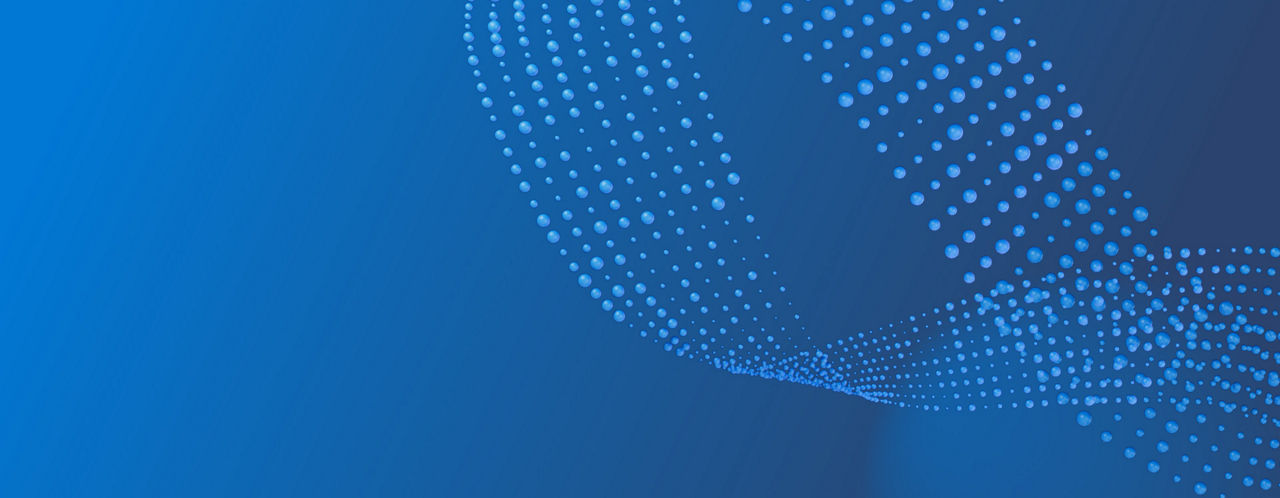# Explore quantum

## What is quantum computing?

Quantum computing holds the promise of solving some of our planet's biggest challenges in the areas of environment, agriculture, health, energy, climate, materials science, and more. For some of these problems, classical computing is increasingly challenged as the size of the system grows. When designed to scale, quantum systems will likely have capabilities that exceed today's most powerful supercomputers. As the global community of quantum researchers, scientists, engineers, and business leaders collaborate to advance the quantum ecosystem, we expect to see quantum impact accelerate across every industry.

Quantum computing uses quantum-mechanical phenomena, such as superposition and entanglement, to perform operations on data. Unlike classical computing, which relies on bits that can be in one of two states (0 or 1), quantum computing uses quantum bits (qubits) that can exist in multiple complex states simultaneously, allowing for parallel computation and quantum interference which can yield exponentially faster calculations.

But a quantum computer isn't a supercomputer that can do everything faster. In fact, one of the goals of quantum computing research is to study which problems can be accelerated by a quantum computer and how large the speedup can be. Quantum computers do exceptionally well with problems that require calculating large numbers of combinations. These types of problems can be found in many areas, such as quantum simulation and cryptography.### Analog and digital quantum computing

Quantum computing is very much an emerging technology and there are several hardware implementations and approaches. Analog and digital quantum computers are two different architectures for implementing quantum computing. The main difference between them is the way they represent and manipulate quantum information.

In analog quantum computing, quantum information is represented by continuous variables, such as the amplitude and phase of a quantum wave function. Analog quantum computing uses a continuous range of values to represent quantum information and manipulates these values using analog circuits and signal processing techniques. Analog quantum computing is often used for problems that involve continuous optimization or simulation.

Digital quantum computing, also known as gate-based quantum computing, uses discrete quantum bits (qubits) to represent and manipulate quantum information using a finite set of values (typically 0 and 1). The quantum information is then manipulated using digital circuits and algorithms. Digital quantum computing uses gates that are similar in concept to logic gates in a classical computer, such the AND-gate and OR-gate. However, unlike a classical computer, a digital quantum computer uses gates to operate on quantum states instead of classical bits. Also, gate-based quantum computers use quantum mechanical systems and their properties, such as the spin of an electron or the polarization of a photon, to represent information.

### Suggested Topics

#### The quantum computing stack

Learn about the layers of the quantum computing stack, from qubits to the high-level programming languages and algorithms used to program a quantum computer

#### The qubit

Learn how qubits use the quantum-mechanical phenomena of superposition and entanglement to power quantum computations

#### Types of qubits

Learn about different qubit implementations, from super conducting, to ion trap, to topological qubits

#### Superposition

Microsoft believes topological qubits are the key to unlocking scaled quantum computing. Learn more about our unique approach